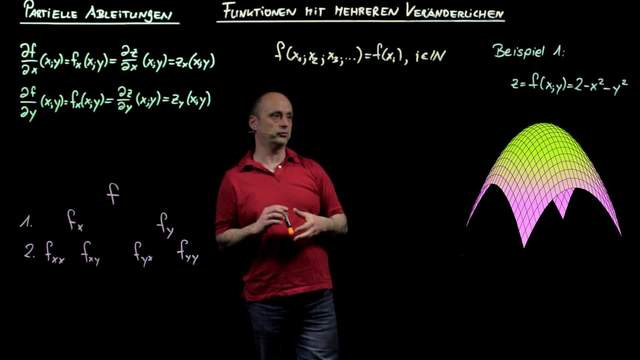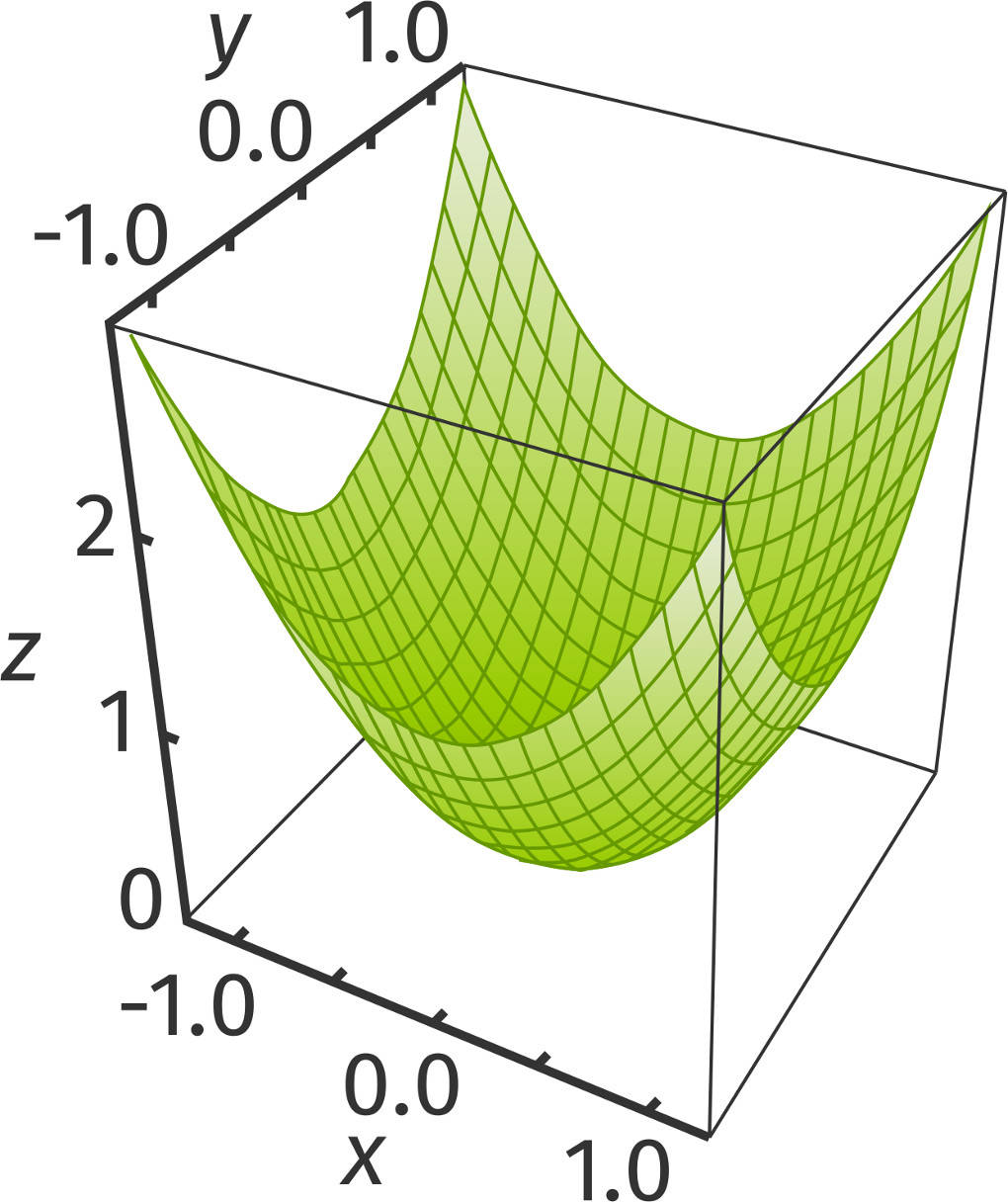# How do you find partial derivatives

## Partial derivative and tangent plane

### Functions with several variables

You already know functions with a variable, for example \$ f (x) = x ^ 2 \$.

For functions with several variables the function depends not only on one variable \$ x \$, but on several: \$ x \$, \$ y \$ and \$ z \$ or - in general - on \$ x_1 \$, \$ x_2 \$, ... We then write a function \$ f \$ like this:

\$ f (x_1; x_2; ...; x_n) \$.

In the following we look at examples of functions with two variables. Everything we learn here can also be applied analogously to more than two variables.Let's start with the function \$ f (x; y) = x ^ 2 + y ^ 2 \$.

The function graph of this function is a Area in the room and is called Paraboloid designated. You can think of this as a 3D parabola.### What is a partial derivative?

If you hold a variable in the function \$ f (x; y) = x ^ 2 + y ^ 2 \$ with \$ y = y_0 \$, you get a function \$ h (x) = f (x; y_0) = x ^ 2 + y_0 ^ 2 \$ in a variable. Its derivative is \$ h '(x) = 2x \$.

So you can have a partial derivative Imagine like this: You derive the function according to one of the two variables and consider the other variable as a constant.

### The first order partial derivatives

The first order partial derivatives are written down as follows:

• \$ \ frac {\ partial f} {\ partial x} = f_x \$ is the partial first order derivative with respect to \$ x \$.
• \$ \ frac {\ partial f} {\ partial y} = f_y \$ is the partial first order derivative with respect to \$ y \$.

The abbreviations \$ f_x \$ and \$ f_y \$ are used more frequently.

The first order partial derivatives can also be written as vectors. This is called gradient designated:

\$ \ nabla f = \ begin {pmatrix} \ frac {\ partial f} {\ partial x} \ \ frac {\ partial f} {\ partial y} \ end {pmatrix} = \ begin {pmatrix} f_x \ f_y \ end {pmatrix} \$.

In the example above, \$ f (x) = x ^ 2 + y ^ 2 \$ is thus \$ f_x = 2x \$ and \$ f_y = 2y \$.

Let's look at another example with the function \$ g (x) = 2 \ sin (x) \ times y-3x \ times \ times y ^ 2 \$. The partial derivatives are:

• \$ g_x = 2 \ cos (x) \ cdot y-3y ^ 2 \$ and
• \$ g_y = 2 \ sin (x) -6x \ cdot y \$.

### The partial derivatives of the second order

As usual, you can also derive functions with several variables several times. The partial derivatives of the second order are formed analogously:

• \$ \ frac {\ partial ^ 2 f} {\ partial ^ 2 x} = f_ {xx} = 2 \$,
• \$ \ frac {\ partial ^ 2 f} {\ partial x ~ \ partial y} = f_ {xy} = 0 \$,
• \$ \ frac {\ partial ^ 2 f} {\ partial y ~ \ partial x} = f_ {yx} = 0 \$ and
• \$ \ frac {\ partial ^ 2 f} {\ partial ^ 2 y} = f_ {yy} = 2 \$.

The partial derivatives of the second order are combined into the so-called Hessian matrix (here in abbreviated form):

\$ \ text {H} _f = \ begin {pmatrix} f_ {xx} & f_ {xy} \ f_ {yx} & f_ {yy} \ end {pmatrix} \$.

For the function \$ f (x; y) = x ^ 2 + y ^ 2 \$ is the Hessian matrix given by this:

\$ \ text {H} _f = \ begin {pmatrix} 2 & 0 \ 0 & 2 \ end {pmatrix} \$.

### Tangent plane at one point

For functions with a variable, you can use the first derivative to set up the equation of a tangent at a point \$ x_0 \$.

This works in a similar way for functions with several variables. Here you put the equation one Tangent plane on.

You need the first order partial derivatives. We look at this again with the example \$ f (x; y) = x ^ 2 + y ^ 2 \$. First we determine the point of contact. In any case, this must fulfill the functional equation: For \$ x_0 = 1 \$ and \$ y_0 = 1 \$ this results in \$ z_0 = f (x_0; y_0) = 1 ^ 2 + 1 ^ 2 = 2 \$. The point to be examined has the coordinates \$ (1 | 1 | 2) \$.

A Tangent plane is generally described by this equation:

\$ z-z_0 = f_x (x_0; y_0) (x-x_0) + f_y (x_0; y_0) (y-y_0) \$.

We can plug our point into this equation:

• This leads to the equation \$ z-2 = 2 (x-1) +2 (y-1) \$.
• You can now transform it as follows: \$ z-2 = 2x-2 + 2y-2 \$, so \$ z-2 = 2x + 2y-4 \$.
• You can convert this equation to a plane equation in coordinate form: \$ E: ~ 2x + 2y-z = 2 \$.

### The necessary condition for extremes

The necessary condition For Extremes for functions with a variable, \$ f '(x) = 0 \$. For functions with several variables, the gradient be the zero vector. This means that every partial first order derivative must be equal to \$ 0 \$. For \$ f (x) = x ^ 2 + y ^ 2 \$ this means:

• \$ f_x = 2x = 0 \$, so \$ x = 0 \$.
• \$ f_y = 2y = 0 \$, so \$ y = 0 \$.

Incidentally, applies as sufficient condition one Hessian matrixthat their Determinant is greater than \$ 0 \$.

• If \$ \ text {H} _ {1; 1} \$ is positive, there is a local minimum in front,
• if \$ \ text {H} _ {1; 1} \$ is negative, there is a local maximum before and

The following properties apply to the function \$ f (x; y) = x ^ 2 + y ^ 2 \$:

• \$ \ det (\ text {H} _f) = 4> 0 \$ and
• \$ \ text {H} _ {1; 1} = 2> 0 \$.

So there is a local minimum, as you can see from the area shown.### The chain rule for functions with several variables

Last but not least, you will learn that Chain rule for functions with several variables know. Let \$ z = f (x (t); y (t)) \$ be a function with several variables. The variables are \$ x \$ and \$ y \$, where both \$ x = x (t) \$ and \$ y = y (t) \$ each depend on the variable \$ t \$.

Then \$ z \$ can be derived from \$ t \$ as follows:

\$ z '(t) = \ frac {\ partial f} {\ partial x} \ cdot x' (t) + \ frac {\ partial f} {\ partial y} \ cdot y '(t) \$.

That sure reminds you of them Chain rule for functions with a variable.

• Here \$ \ frac {\ partial f} {\ partial x} \$ and \$ \ frac {\ partial f} {\ partial y} \$ are the derivatives of the external functions.
• \$ x '(t) = \ frac {dx} {dt} \$ and \$ y' (t) = \ frac {dy} {dt} \$ are the derivatives of the inner functions.

Again, let's look at an example:

\$ z (t) = (x (t)) ^ 2+ (y (t)) ^ 2 = (t ^ 2-2) ^ 2 + (2t + 1) ^ 2 \$.

Here \$ x (t) = t ^ 2-2 \$ and thus the first derivative \$ x '(t) = 2t \$ as well as \$ y (t) = 2t + 1 \$ and the first derivative \$ y' (t) = \$ 2.

Now the chain rule can be applied:

\$ \ begin {array} {rcl} z '(t) & = & 2x (t) \ times 2t + 2y (t) \ times 2 \ & = & 2 (t ^ 2-2) \ times 2t + 2 (2t +1) \ cdot 2 \ & = & 4t ^ 3-8t + 8t + 4 \ & = & 4t ^ 3 + 4. \ end {array} \$